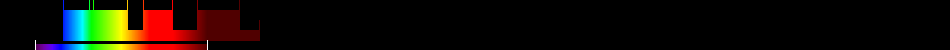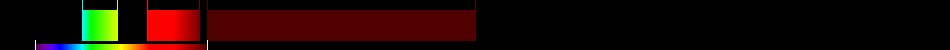# Information for Sensor and Index

## Formula

General Formula $\frac{\frac{{\mathrm{NIR}}}{{\mathrm{NIR}}+{\mathrm{RED}}}}{2}\left({\mathrm{NDVI}}+1\right)$ $\frac{\frac{{\mathrm{nahe_Infrarot}}}{{\mathrm{nahe_Infrarot}}+{\mathrm{Rot}}}}{2}\left(\left(\frac{{\mathrm{Rot}}-{\mathrm{GrÃ¼n}}}{{\mathrm{Rot}}+{\mathrm{GrÃ¼n}}}\right)+1\right)$ Automatic

## Sensor

Name IKONOS-2 5 445-900 0.82-3.2 98.1 GeoEye 1999-09-24 original IKONOS-1 was lost during launch at 1999-04-27 yes

## Index

Name Infrared percentage vegetation index IPVI $\frac{\frac{{\mathrm{NIR}}}{{\mathrm{NIR}}+{\mathrm{RED}}}}{2}\left({\mathrm{NDVI}}+1\right)$ 490:570,640:760,780:1400 Original formula

## References